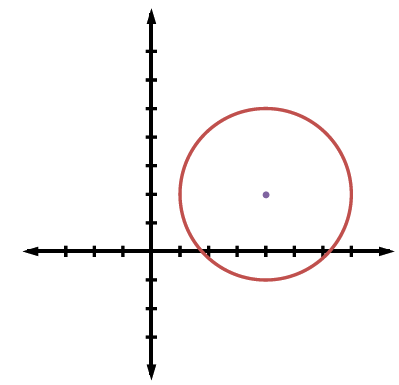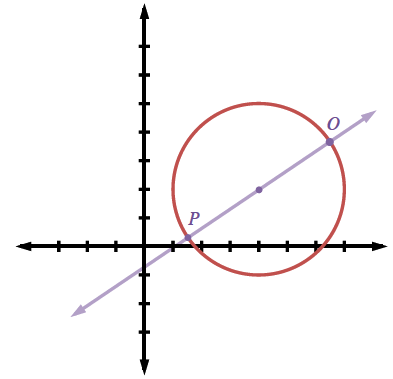### Home > CCG > Chapter 12 > Lesson 12.1.2 > Problem12-31

12-31.

On graph paper, graph a circle with center $\left(4,2\right)$ and radius $3$ units.

1. Write its equation.

$(x − 4)^2 + (y − 2)^2 = 9$2. Find two points on the circle that are on opposite sides of the circle, that is they are $180^\circ$ from one another. Prove that these two points are exactly one diameter in distance from each other.

Draw a line through the center of the circle to find two points that are $180^\circ$ from one another.Find the distance between point $O$ and point $P$.